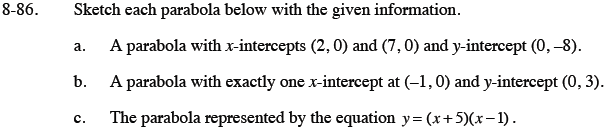### Home > CCA > Chapter 8 > Lesson 8.2.3 > Problem8-86

8-86.
1. Sketch each parabola below with the given information. 8-86 HW eTool (Desmos). Homework Help ✎

1. A parabola with x-intercepts (2, 0) and (7, 0) and y-intercept (0, −8).

2. A parabola with exactly one x-intercept at (−1, 0) and y-intercept (0, 3).

3. The parabola represented by the equation y = (x + 5)(x − 1).Use the eTool below to help sketch each of the parabolas.
Click on the link at right for the full eTool version: CCA 8-86 HW eTool